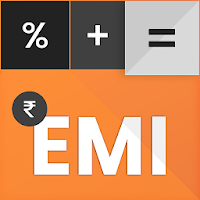EMI Calculator Pro - Easy Home Loan Calculator# EMI Calculator Pro - Easy Home Loan Calculator

## Salary loan calculator, loan EMI, EMI alerts,Loan Calculator, Interest Rate

Developer: VI Games
App Size: 14M
Release Date: Apr 11, 2019
Price: Free
4.7
7 Ratings
Size
14M

### Screenshots for App

Mobile
EMI Calculator Pro - Easy Home Loan Calculator is a simple loan calculation tool that helps the user to quickly calculate EMI and view loan Affordability and also you can compare two loans. Use this app to calculate your EMI (Equated Monthly Instalment), plan your loan repayment effective way.

FEATURES:
● EMI Calculator Pro - Easy Home Loan Calculator is the special kind of calculator that calculates your Loan EMI.
● EMI Calculator Pro - Easy Home Loan Calculator app allows you to calculate the following values by inputting all the other values:
- EMI
- Loan Amount
- Interest Rate
- Period (In Months and Years)
● Representation of payment split up in the table form.
● Calculate EMI on a monthly basis.
● Generate statistics chart instantly.
● Statistics show Principal Amount, Interest rate and remaining balance per month.
● Share computed PDF with anyone for EMI & loan planning.

USAGES:
● Loan Calculator
● EMI Calculator
● Standard EMI Calculator
● EMI Statistics
● Finance Calculator & Statistics